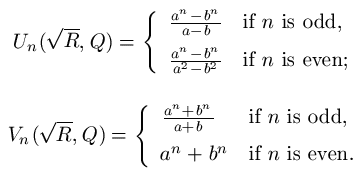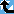# Lehmer number

The Prime Pages keeps a list of the 5000 largest known primes, plus a few each of certain selected archivable forms and classes. These forms are defined in this collection's home page.

Lucas generalized the sequence of Fibonacci numbers as follows: let a and b be the zeros of the polynomial x2-Px+Q (where P, Q and D = P2-4Q are non-zero integers), then set
Un(P,Q) = (an - bn)/(a - b),     and     Vn(P,Q) = an + bn.
Lehmer noted that we can loosen the restriction that P be an integer and still get a sequence of integers by replacing P with the squareroot of R and slightly modifying these definitions.  To make sure the sequences are not zero infinitely often we require that a/b not be a root of unity:These share many properties with the generalized Lucas numbers.

For integers P, the two numbers Uk+1(P,1) ± Uk(P,1) are Lehmer numbers whose product is equal to U2k+1(P,1).  This follows from the fact that when Q=1 we may write (P ± sqrt(P2-4Q))/2 as the square of (sqrt(P+2) ± sqrt(P-2))/2, and hence obtain Lehmer's sqrt(R) as sqrt(P+2).

Note that the Lehmer numbers Uk+1(P,1) ± Uk(P,1) cannot be prime if 2k+1 is composite.

###Record Primes of this Type

rankprime digitswhowhencomment
1U(15694, 1, 14700) + U(15694, 1, 14699) 61674 x45 Aug 2019 Lehmer number
2U(809, 1, 17325) - U(809, 1, 17324) 50378 x45 Jul 2019 Lehmer number
3U(52245, 1, 9241) + U(52245, 1, 9240) 43595 x45 Jul 2019 Lehmer number
4U(35896, 1, 7260) + U(35896, 1, 7259) 33066 x45 Jul 2019 Lehmer number
5U(23396, 1, 6615) + U(23396, 1, 6614) 28898 x45 Jul 2019 Lehmer number
6U(16531, 1, 6721) - U(16531, 1, 6720) 28347 x36 May 2007 Lehmer number
7U(5092, 1, 7561) + U(5092, 1, 7560) 28025 x25 Oct 2014 Lehmer number
8U(5239, 1, 7350) - U(5239, 1, 7349) 27333 CH10 Jun 2017 Lehmer number
9U(1766, 1, 7561) - U(1766, 1, 7560) 24548 x25 Nov 2013 Lehmer number
10U(1383, 1, 7561) + U(1383, 1, 7560) 23745 x25 Nov 2013 Lehmer number
11U(1118, 1, 7561) - U(1118, 1, 7560) 23047 x25 Oct 2013 Lehmer number
12U(43100, 1, 4620) + U(43100, 1, 4619) 21407 x25 Jun 2016 Lehmer number
13U(15631, 1, 5040) - U(15631, 1, 5039) 21134 x25 Apr 2003 Lehmer number
14U(35759, 1, 4620) + U(35759, 1, 4619) 21033 x25 May 2016 Lehmer number
15U(31321, 1, 4620) - U(31321, 1, 4619) 20767 x25 Jun 2016 Lehmer number
16U(22098, 1, 4620) + U(22098, 1, 4619) 20067 x25 Jun 2016 Lehmer number
17U(21412, 1, 4620) - U(21412, 1, 4619) 20004 x25 Jun 2016 Lehmer number
18U(19361, 1, 4620) + U(19361, 1, 4619) 19802 x25 May 2016 Lehmer number
19U(9657, 1, 4321) - U(9657, 1, 4320) 17215 x23 Dec 2005 Lehmer number
20U(15823, 1, 3960) - U(15823, 1, 3959) 16625 x25 Nov 2002 Lehmer number, cyclotomy

###References

Gyory1982
Györy, K., "On some arithmetical properties of Lucas and Lehmer numbers," Acta Arith., 40:4 (1981/82) 369--373.  MR667047
Gyory2003
Györy, K., "On some arithmetical properties of Lucas and Lehmer numbers. II," Acta Acad. Paedagog. Agriensis Sect. Mat. (N.S.), 30 (2003) 67--73.  Dedicated to the memory of Professor Dr. P\'eter Kiss.  MR2054716
LP2003
Luca, F. and Porubský, S., "The multiplicative group generated by the Lehmer numbers," Fibonacci Quart., 41:2 (2003) 122--132.  MR1990520
McDaniel1993
McDaniel, W., "Square Lehmer numbers," Colloq. Math., 66:1 (1993) 85--93.  MR1242648
Ribenboim95
P. Ribenboim, The new book of prime number records, 3rd edition, Springer-Verlag, New York, NY, 1995.  pp. xxiv+541, ISBN 0-387-94457-5. MR 96k:11112 [An excellent resource for those with some college mathematics. Basically a Guinness Book of World Records for primes with much of the relevant mathematics. The extensive bibliography is seventy-five pages.]
RW1980
Rotkiewicz, A. and Wasén, R., "Lehmer's numbers," Acta Arith., 36:3 (1980) 203--217.  MR581371
Schinzel1962
Schinzel, A., "On primitive prime factors of Lehmer numbers. I," Acta. Arith., 8 (1962/1963) 213--223.  MR 27:1408
Schinzel1962b
Schinzel, A., "The intrinsic divisors of Lehmer numbers in the case of negative discriminant," Ark. Mat., 4 (1962) 413--416 (1962).  MR0139567
Schinzel1963
Schinzel, A., "On primitive prime factors of Lehmer numbers. II," Acta. Arith., 8 (1962/1963) 251--257.  MR 27:1409
Schinzel1968
Schinzel, A., "On primitive prime factors of Lehmer numbers. III," Acta Arith., 15 (1968) 49--70.  MR0232744
SS1981
Shorey, T. N. and Stewart, C. L., "On divisors of Fermat, Fibonacci, Lucas and Lehmer numbers. II," J. London Math. Soc. (2), 23:1 (1981) 17--23.  MR 82m:10025
Stewart1976
Stewart, C. L., Primitive divisors of Lucas and Lehmer numbers.  In "Transcendence theory: advances and applications (Proc. Conf., Univ. Cambridge, Cambridge, 1976)," Academic Press, 1977.  London, pp. 79--92, MR0476628
Stewart1977
C. L. Stewart, "On divisors of Fermat, Fibonacci, Lucas and Lehmer numbers," Proc. Lond. Math. Soc., 35:3 (1977) 425--447.  MR 58:10694
Stewart1983
Stewart, C. L., "On divisors of Fermat, Fibonacci, Lucas and Lehmer numbers. III," J. London Math. Soc. (2), 28:2 (1983) 211--217.  MR 85g:11021
Ward1955
Ward, M., "The intrinsic divisors of Lehmer numbers," Ann. of Math. (2), 62 (1955) 230--236.  MR0071446